# 卷积神经网络

Posted by Masutangu on September 4, 2022

# 从全连接层到卷积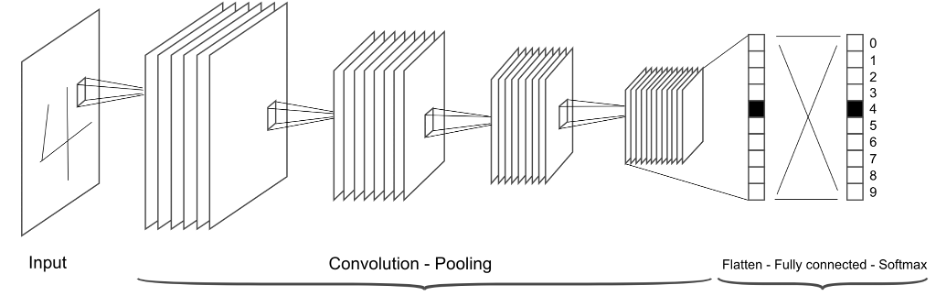$(f * g)(\mathbf{x}) = \int f(\mathbf{z}) g(\mathbf{x}-\mathbf{z}) d\mathbf{z}$

$(f * g)(i) = \sum_a f(a) g(i-a)$

$(f * g)(i, j) = \sum_a\sum_b f(a, b) g(i-a, j-b)$

$[\mathbf{H}]_{i, j} = u + \sum_{a = -\Delta}^{\Delta} \sum_{b = -\Delta}^{\Delta} [\mathbf{V}]_{a, b} [\mathbf{X}]_{i+a, j+b}$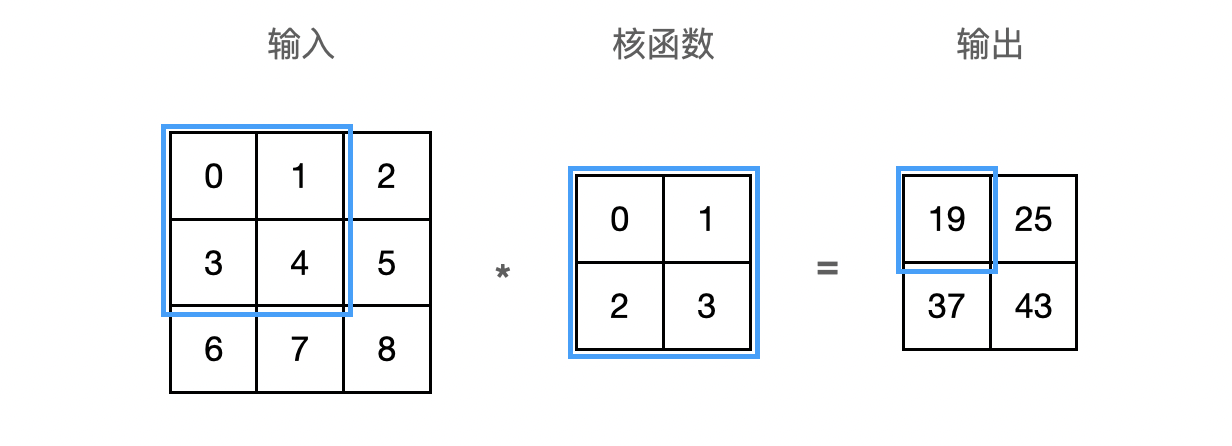$0\times0+1\times1+3\times2+4\times3=19$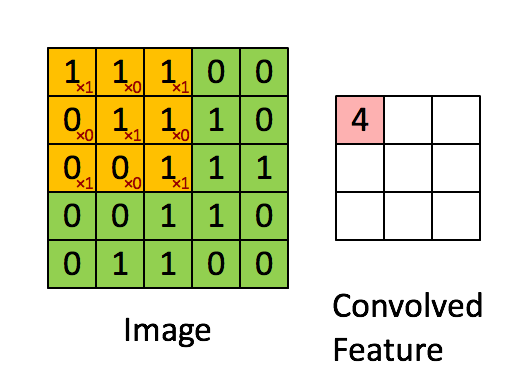## 局部感知野 Local Receptive Field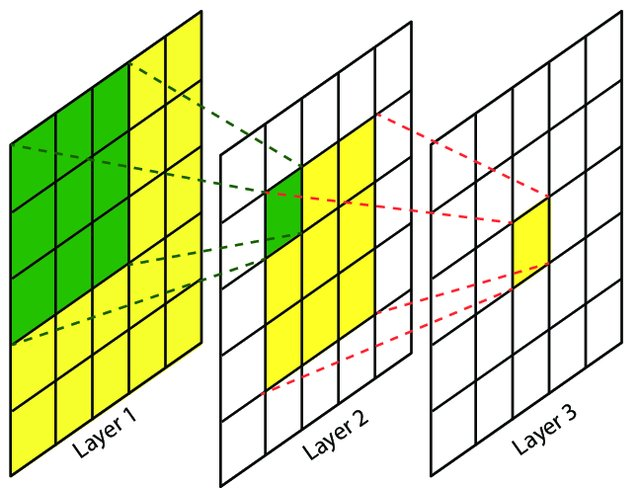## 权值共享# 填充和步幅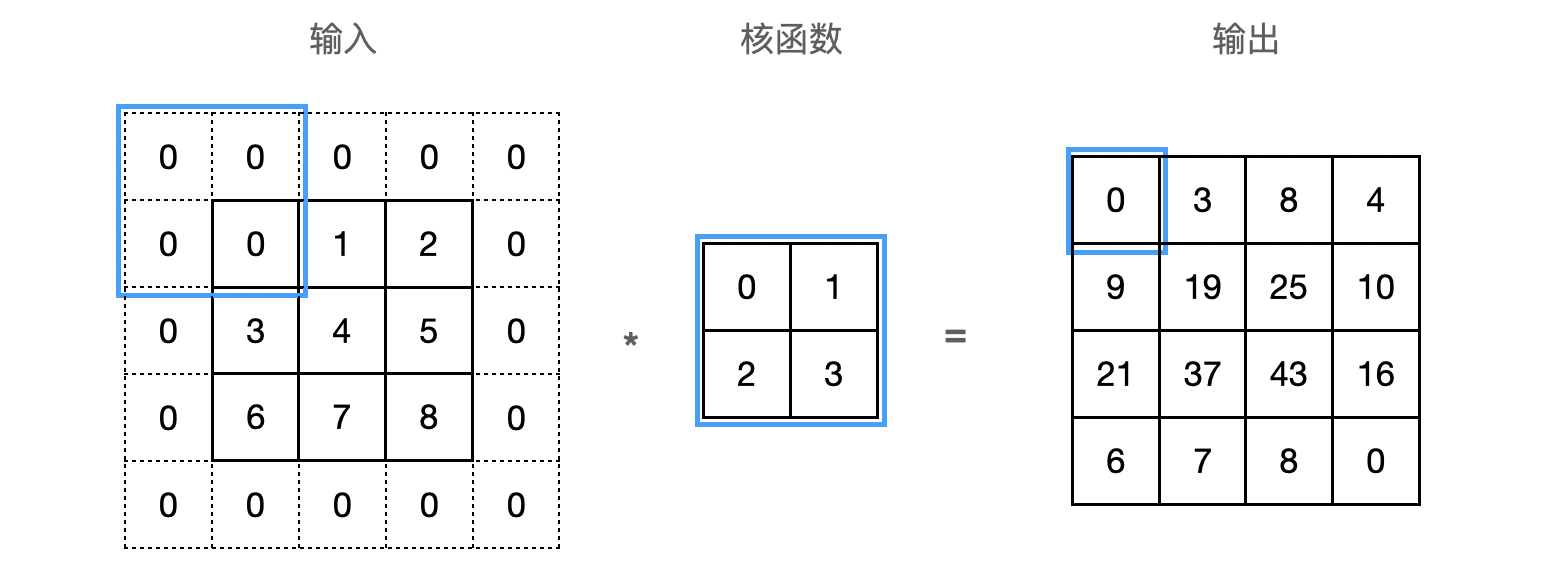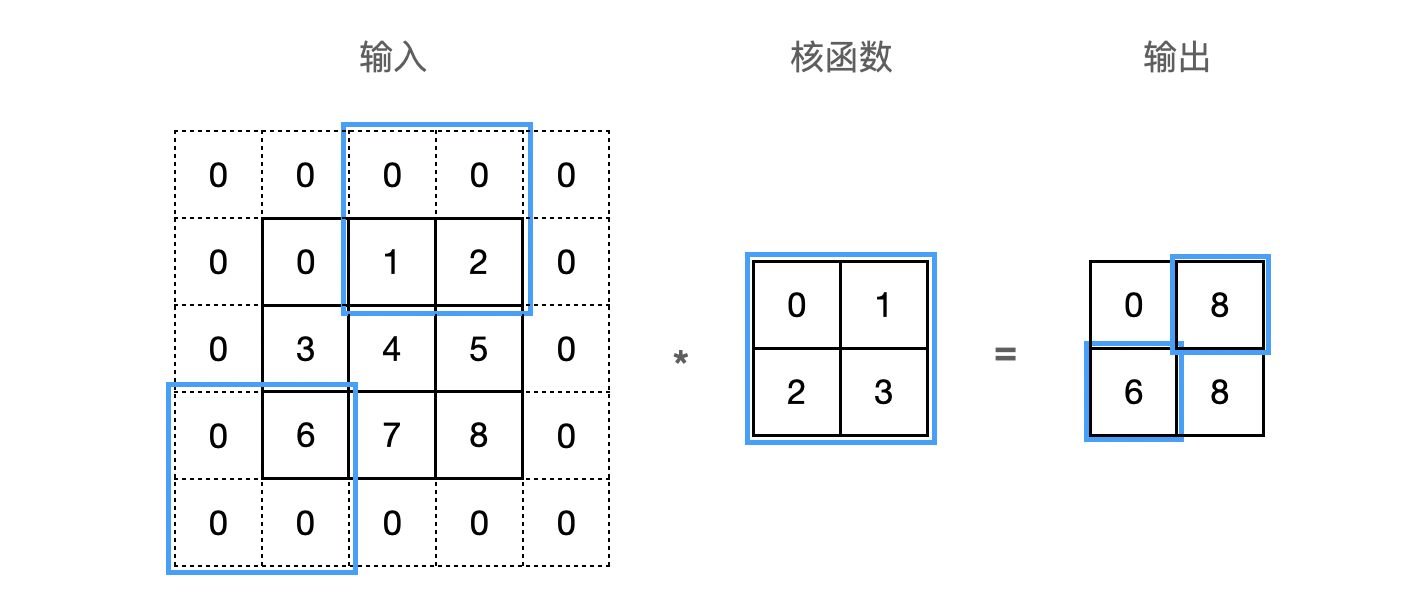# 多输入多输出通道

### 多输入通道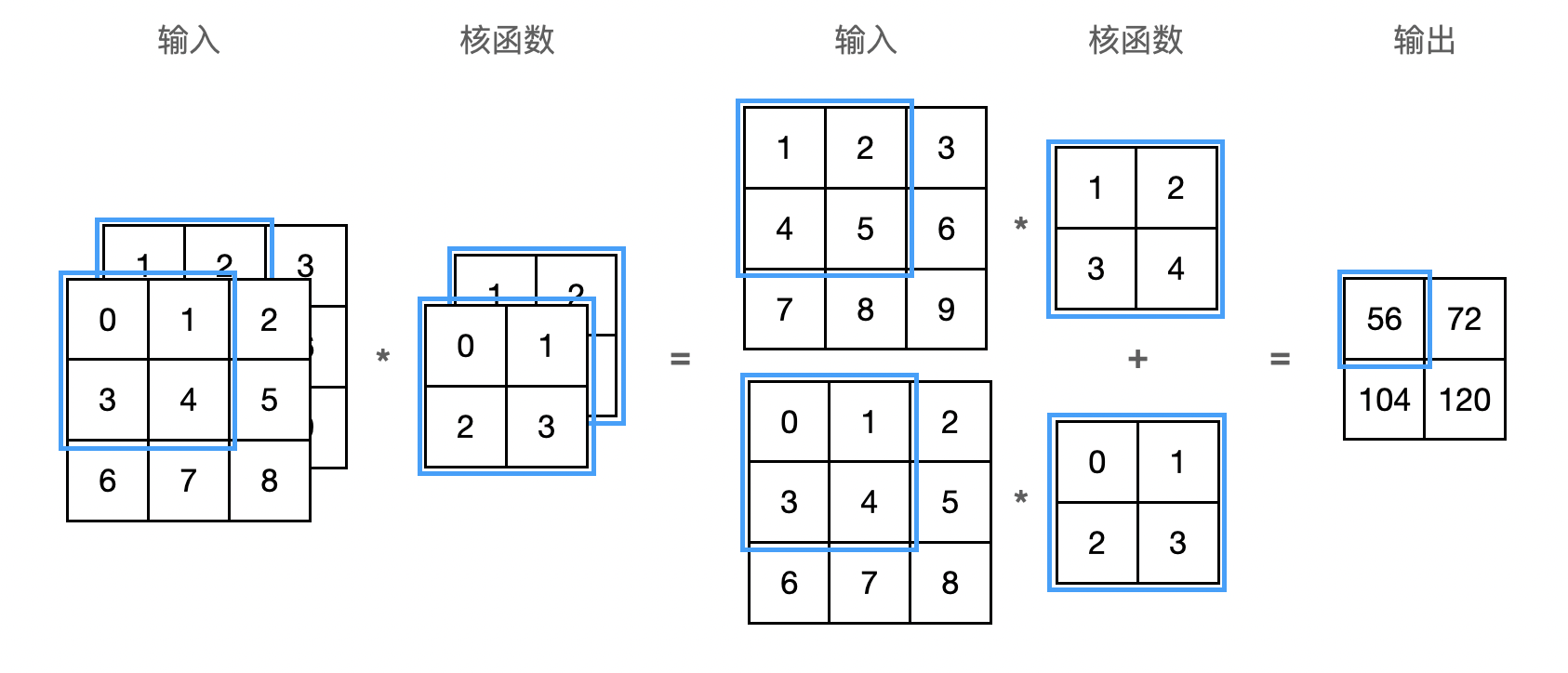## $1 \times 1$ 卷积层

$1 \times 1$ 卷积，即 $k_h = k_w = 1$，看起来似乎没有多大意义，但十分流行，经常包含在复杂深层网络的设计中。使用 $1 \times 1$ 窗口，就失去了卷积层的特有能力——在高度和宽度维度上，识别相邻元素间相互作用的能力。 其实 $1 \times 1$ 卷积的唯一计算发生在通道上。我们可以将 $1 \times 1$ 卷积层看作是在每个像素位置应用的全连接层，以 $c_i$ 个输入值转换为 $c_o$ 个输出值：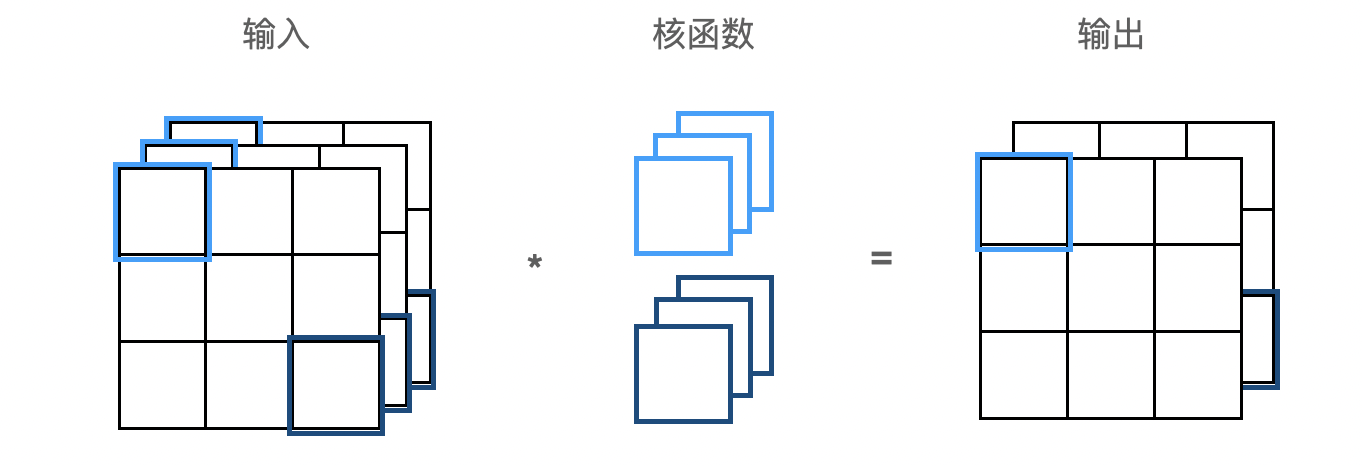# 汇聚层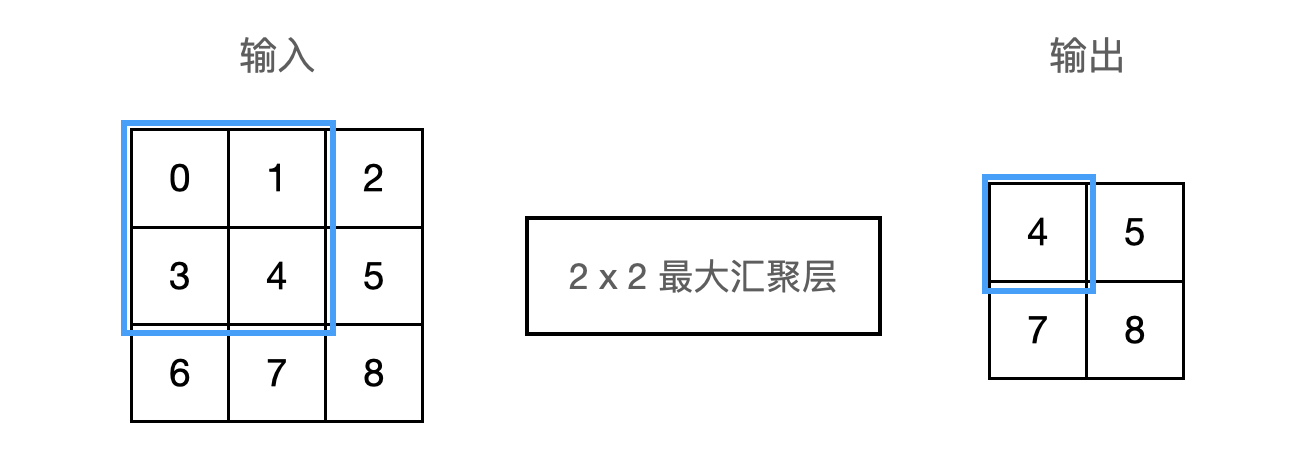# 参考文献